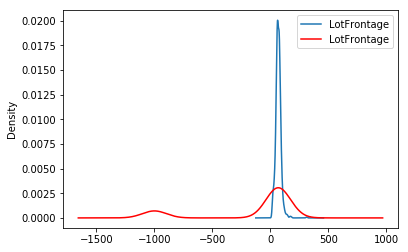# ArbitraryNumberImputer#

The `ArbitraryNumberImputer()` replaces missing data with an arbitrary numerical value determined by the user. It works only with numerical variables.

The `ArbitraryNumberImputer()` can find and impute all numerical variables automatically. Alternatively, you can pass a list of the variables you want to impute to the `variables` parameter.

You can impute all variables with the same number, in which case you need to define the variables to impute in the `variables` parameter and the imputation number in `arbitrary_number` parameter. For example, you can impute varA and varB with 99 like this:

```transformer = ArbitraryNumberImputer(
variables = ['varA', 'varB'],
arbitrary_number = 99
)

Xt = transformer.fit_transform(X)
```

You can also impute different variables with different numbers. To do this, you need to pass a dictionary with the variable names and the numbers to use for their imputation to the `imputer_dict` parameter. For example, you can impute varA with 1 and varB with 99 like this:

```transformer = ArbitraryNumberImputer(
imputer_dict = {'varA' : 1, 'varB': 99}
)

Xt = transformer.fit_transform(X)
```

Below a code example using the House Prices Dataset (more details about the dataset here).

First, let’s load the data and separate it into train and test:

```import numpy as np
import pandas as pd
import matplotlib.pyplot as plt
from sklearn.model_selection import train_test_split

from feature_engine.imputation import ArbitraryNumberImputer

# Separate into train and test sets
X_train, X_test, y_train, y_test = train_test_split(
data.drop(['Id', 'SalePrice'], axis=1),
data['SalePrice'],
test_size=0.3,
random_state=0,
)
```

Now we set up the `ArbitraryNumberImputer()` to impute 2 variables from the dataset with the number -999:

```# set up the imputer
arbitrary_imputer = ArbitraryNumberImputer(
arbitrary_number=-999,
variables=['LotFrontage', 'MasVnrArea'],
)

# fit the imputer
arbitrary_imputer.fit(X_train)
```

With `fit()`, the transformer does not learn any parameter. It just assigns the imputation values to each variable, which can be found in the attribute `imputer_dict_`.

With transform, we replace the missing data with the arbitrary values both in train and test sets:

```# transform the data
train_t= arbitrary_imputer.transform(X_train)
test_t= arbitrary_imputer.transform(X_test)
```

Note that after the imputation, if the percentage of missing values is relatively big, the variable distribution will differ from the original one (in red the imputed variable):

```fig = plt.figure()In the following Jupyter notebook you will find more details on the functionality of the `ArbitraryNumberImputer()`, including how to select numerical variables automatically. You will also see how to navigate the different attributes of the transformer.